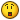## Recommended Posts

I was just wondering how do you "fill" up an array with different values?

I tried something simple just to try get the idea.

```#include <array.au3>

\$as_test = ''

For \$x = 1 To 100
\$as_test = \$x
Next

_ArrayDisplay(\$as_test, "test")```

The above doesnt work at all, what i tried to do was to fill \$as_test with values, saving them to an "array". But this isnt how it works right?

"I'm paper, rock is fine, nerf scissors!!!"

##### Share on other sites

```#include <array.au3>

Dim \$as_test

For \$x = 1 To 10
\$as_test[\$x] = \$x
Next

_ArrayDisplay(\$as_test, "test")```

Live for the present,
Dream of the future,
Learn from the past.##### Share on other sites

```#include <array.au3>

Dim \$as_test

For \$x = 1 To 10
\$as_test[\$x] = \$x
Next

_ArrayDisplay(\$as_test, "test")```
Ye i kinda figured you would use the \$as_test, as you know how many variables will be saved into the array. I missed that out in the question sorry, lets assume we have no idea how many values will be put into the array"I'm paper, rock is fine, nerf scissors!!!"

##### Share on other sites

I was just wondering how do you "fill" up an array with different values?

I tried something simple just to try get the idea.

```#include <array.au3>

\$as_test = ''

For \$x = 1 To 100
\$as_test = \$x
Next

_ArrayDisplay(\$as_test, "test")```

The above doesnt work at all, what i tried to do was to fill \$as_test with values, saving them to an "array". But this isnt how it works right?

close, but you're just re-using the same value. you have to have alist referenced by index number, etc like:

```\$as_test = 1
\$as_test = 2```

for your example, try something like:

```#include<array.au3>; you have to include array.au3 to use _arraydisplay()
dim \$as_test; the 100 means there will be 100 elements, from 0 - 99
\$as_test=0
for \$x = 1 to 99; autoit convention is to put the number of indexes into the 0th element, so start filling at 1
\$as_test[\$x] = \$x
\$as_test = \$as_test + 1
next
_ArrayDisplay(\$as_test,"Test Array")```

##### Share on other sites

Thanks for the fast responses, but i left out the thing "i dont know how many values will be added into the array". In the above examples we know how many values are to be added, but if that is unknown what is the trick?Lets say ill go thru a file and want to save each line into an array. Since i dont know how many lines the file has, how would i then do? (im using a file as an example, but it could be anything really).

Edited by huldu

"I'm paper, rock is fine, nerf scissors!!!"

##### Share on other sites

Don't argue with an idiot; people watching may not be able to tell the difference.

##### Share on other sites

Thank you, was just something like that i were looking for"I'm paper, rock is fine, nerf scissors!!!"

##### Share on other sites

Thanks for the fast responses, but i left out the thing "i dont know how many values will be added into the array". In the above examples we know how many values are to be added, but if that is unknown what is the trick?you can redim if necessary per iteration, OR if the array is being returned by a function like FileReadToArray() or StringSplit() then don't give it a dimension when you declare it...

example

```#include<array.au3>
dim \$as_test
\$as_test = StringSplit("this,is,a,comma,delimited,string,that,will,be,in,your,array",",")

## Create an account

Register a new account

• ### Recently Browsing   0 members

×

• Wiki

• Back

• #### Beta

• Git
• FAQ
• Our Picks
×
• Create New...